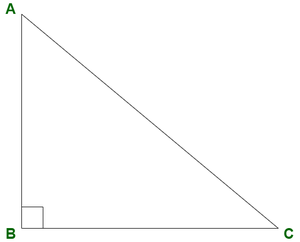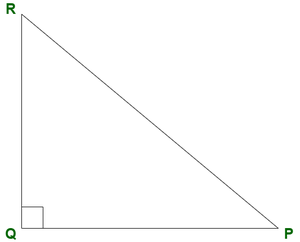Find the value of cot(90° –θ) cosec(90° –θ) sinθ tanθ tan(90° –θ)

• Last Updated : 05 Oct, 2021

Trigonometry is derived from Latin words, ‘trigonon’ meaning triangle and ‘metron’ meaning measure, which simply means measurement of triangles. But what is meant by measuring triangles? As it is known that a triangle has 3 sides and 3 angles. Trigonometry studies the relationship between these 3 sides and 3 angles.

Trigonometry has applications in a wide variety of fields like astronomy, architecture, satellite navigation system, land surveying, etc. Trigonometry finds itself in various natural phenomena like periodic motions, simple harmonic motions, quantum mechanics, optics and acoustics, etc. Trigonometry can be applied to any type of triangle but for the scope of this article, we will limit ourselves to the right-angled triangle. Let’s look at the foundation of trigonometry.

Trigonometric Ratios

Consider a right-angled triangle ABC shown below. Let us consider for a moment ∠C. Before understanding trigonometric ratios one has to understand 3 terms associated with the angle which we have considered (here, it is ∠C.)• Perpendicular The side opposite to the angle in consideration is called perpendicular. In our diagram, perpendicular to ∠C is AB.
• Hypotenuse The side opposite to the right angle is called the hypotenuse. In the above diagram, the hypotenuse is AC.
• Base The side adjacent to the angle in consideration is called the base. In the above diagram, for ∠C, the base in BC.

Now it is understood the meaning of perpendicular, hypotenuse, and the base, let’s move further. Since there are three sides in ∆ABC, take any two sides to form a ratio. Applying permutations for three sides, calculate that form six ratios. Each of these six ratios is given a special name.

1. sine of ∠C = sin C = Perpendicular/Hypotenuse = AB/AC
2. cosecant of ∠C = cosec C = Hypotenuse/Perpendicular = AC/AB
3. cosine of ∠C = cos C = Base/Hypotenuse = BC/AC
4. secant of ∠C = sec C = Hypotenuse/Base = AC/BC
5. tangent of ∠C = tan C = Perpendicular/Base = AB/BC
6. cotangent of ∠C = cot C = Base/Perpendicular = BC/AB

Reciprocal Relationship

As we have seen above that sin C = AB/AC  and cosec C =  AC/AB. Here, observe that sin and cosec are reciprocal of each other. Therefore, the following are the reciprocal relations between the trigonometric ratios, For all angle θ,

• sinθ = 1/cosecθ  and cosecθ = 1/sinθ
• cosθ = 1/secθ  and  secθ = 1/cosθ
• tanθ = 1/cotθ and cotθ = 1/tanθ

Also, formulae for tanθ and cotθ.

• tanθ = sinθ/cosθ
• cotθ = cosθ/sinθ

Trigonometric Identities

The six trigonometric ratios are connected to each other by three trigonometric identities which are as follows,

• sin2θ + cos2θ = 1
• sec2θ – tan2θ = 1
• cosec2θ – cot2θ = 1

Complementary Angles and Ratios

Let’s go back to learn complementary angles. Two angles are said to be complementary if their sum is 90°. Consider ∆QPR is given below, right-angled at Q. Similarly, two angles are said to be supplementary if their sum is 180°.On applying angle sum property, ∠P + ∠Q + ∠R = 180°

∠P + 90° + ∠R = 180°

∠P + ∠R = 90°

So, the sum of ∠P and ∠Q is 90°. This is true for any right-angled triangle in that two angles besides the right angle are always complimentary. Remember this point as this will be useful in further discussion. Now consider sin P = RQ/RP and cos R = RQ/RP. Here notice that sin P = cos R. Similarly, consider tan P = RQ/QP and cot R = RQ/QP. Here, see that tan P = cot R.

Remember that ∠P and ∠R are complementary angles and the sine of one is equal to the cosine of the other. Hence, concluded that sine and cosine are complementary of each other, i.e. they are complementary ratios. Similarly, tan and cot are complementary and cosecant and secant are complementary ratios. So, the following results are obtained, If θ and (90° – θ) are two complementary angles then,

• sin(90° – θ) = cos θ
• cosec(90° – θ) = sec θ
• tan(90° – θ) = cot θ
• cos(90° – θ) = sin θ
• sec(90° – θ) = cosec θ
• cot(90° – θ) = tan θ

Now let’s look at some sample problems related to the formulae learned above.

Find the value of cot(90° – θ) cosec(90° – θ) sinθ tanθ tan(90° – θ)

Formulae which will be used for solving this question

1. cot(90° – θ) = tan θ
2. cosec(90° – θ) = sec θ
3. tan(90° – θ) = cot θ
4. cot θ × tan θ = 1
5. tan θ = sinθ/cosθ
6. sec θ = 1/cosθ

Solution:

Consider the question cot(90 – θ) cosec(90 – θ) sin θ tan θ tan(90 – θ). Using the above formulae 1, 2 and 3, convert the expression as follows: tan θ sec θ sin θ tan θ cot θ.

• Now using formula 4, modifying the last two terms of first step, tan θ sec θ sin θ × 1.
• Using formula 5, the expression becomes: sinθ/cosθ × sec θ × sin θ.
• Applying formula 6, the expression is converted to: sinθ/cosθ × 1/cosθ  × sin θ

Next step is to simplify: (sinθ × sinθ) / (cosθ × cosθ) = sin2θ / cos2θ

Now, sinθ/cosθ = tan θ (formula 5),

Therefore, sin2θ / cos2θ = tan2θ

So, final value of cot(90 – θ) cosec(90 – θ) sin θ tan θ tan(90 – θ) is tan2θ.

Similar Problems

Question 1: Find the value of (tan(90° – θ) cosecθ cos(90° – θ)) / (sec(90° – θ) sin(90° – θ) cosθ)

Solution:

Consider the problem (tan(90° – θ) cosecθ cos(90° – θ)) / (sec(90° – θ) sin(90° – θ) cosθ)

On using the formulae,

• tan( 90° – θ) = cot θ,
• cos( 90° – θ) = sin θ,
• sec( 90° – θ) = cosecθ, and
• sin( 90° – θ) = cos θ

We get (cotθ cosecθ sinθ) / (cosecθ cosθ cosθ)

On further solving, (cotθ sinθ) / (cos2θ)

Now using the formula cotθ = cosθ/sinθ,

= (cosθ sinθ)/(sinθ cos2θ)

Finally, 1/cosθ which is equal to secθ by reciprocal relation.

So, (tan(90° – θ) cosecθ cos(90° – θ)) / (sec(90° – θ) sin(90° – θ) cosθ) = secθ

Question 2: Evaluate the following: tan(90° – θ) secθ – cot(90° – θ) cosθ / cos2 (90° – θ)

Solution:

On using the formulae

• tan(90° – θ) = cotθ,
• cot(90° – θ) = tanθ and
• cos2(90° – θ) = sin2θ,

The problem modifies to cotθ.secθ – tanθ(cosθ / sin2θ)

Using the formulae,

• cotθ = cosθ/sinθ,
• secθ = 1/cosθ and
• tanθ = sinθ/cosθ

= (cosθ/sinθ)(1/cosθ) – (sinθ / cosθ).cosθ/(sin2θ)

On solving further, 1/sinθ – 1/sinθ = 0

So, tan(90° – θ)secθ – cot(90° – θ)cosθ/cos2(90° – θ) = 0

My Personal Notes arrow_drop_up